#### Howdy, Stranger!

It looks like you're new here. If you want to get involved, click one of these buttons!

Options

# Lecture 50 - Chapter 3: Left Kan Extensions

edited February 2020

Okay, let's see some examples! We'll create new databases from old using Kan extensions. You can see Fong and Spivak doing this in Section 3.4 of Seven Sketches, but I'll warn you that they don't say "Kan extension". They call the left Kan extension $$\sum$$ and the right Kan extension $$\prod$$, for reasons that will eventually become clear. (All mathematical concepts are connected.)

We'll start with the easiest example that's really nice. As a database schema let's use the free category on this graph: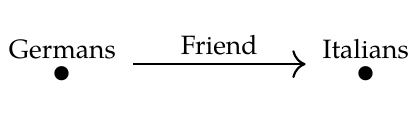Let's call this category $$\mathcal{C}$$. A functor $$F: \mathcal{C} \to \mathbf{Set}$$ is a database consisting of a set of Germans, a set of Italians, and a function mapping each German to an Italian. We can present it in table form, like this: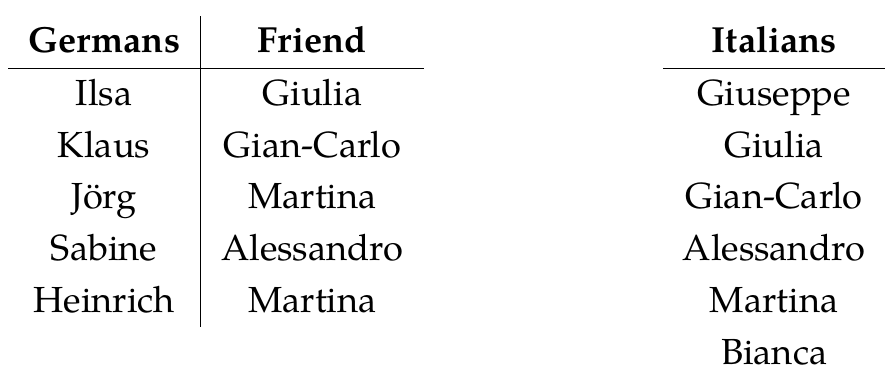Now suppose we want to forget all the information involving Italians. For this we need a simpler database schema, namely the free category on this graph: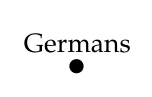Let's call this category $$\mathcal{D}$$. A functor $$F: \mathcal{D} \to \mathbf{Set}$$ is a database consisting of a set of Germans.

There's a unique functor

$$G: \mathcal{D} \to \mathcal{C}$$ with the property that

$$G(\mathrm{Germans}) = \mathrm{Germans} .$$ Any functor $$F: \mathcal{C} \to \mathbf{Set}$$ can be composed with $$G$$ to give a functor $$F \circ G : \mathcal{D} \to \mathbf{Set}$$. This process has the effect of forgetting the Italians!

So, if $$F$$ was this database:then $$F \circ G$$ is this database: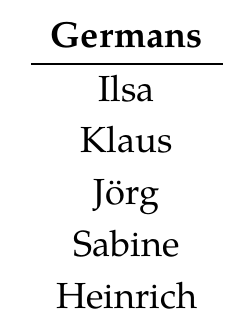Okay, that was pretty simple I hope! To put it in fancier terms, I just showed you what the functor

$$\textrm{ composing with } G : \mathbf{Set}^{\mathcal{C}} \to \mathbf{Set}^{\mathcal{D}}$$ does to objects - that is, databases. Now let's try to reverse this process, which is the fun part. Let's take a database that's just a set of Germans and try to turn it into a database that also includes Italians, and an Italian friend for every German!

Of course this is a bit odd, because we're trying to recover data that can't really be recovered. But that's exactly what adjoints are for.

There are two ways to proceed: the left adjoint way, called

$$\textrm{Lan}_G : \mathbf{Set}^{\mathcal{D}} \to \mathbf{Set}^{\mathcal{C}}$$ and the right adjoint way, called

$$\textrm{Ran}_G : \mathbf{Set}^{\mathcal{D}} \to \mathbf{Set}^{\mathcal{C}}$$ As usual, the left adjoint is 'liberal' or 'generous'. It says "We have to give each German an Italian friend? Okay, let's give them each their own friend!"

So, if we start with a database $$H : \mathcal{D} \to \mathbf{Set}$$ like this: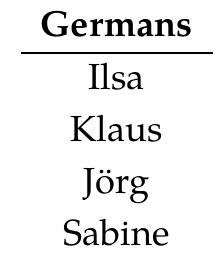then the left adjoint method gives us this database $$\textrm{Lan}_G(H) : \mathcal{C} \to \mathbf{Set}$$: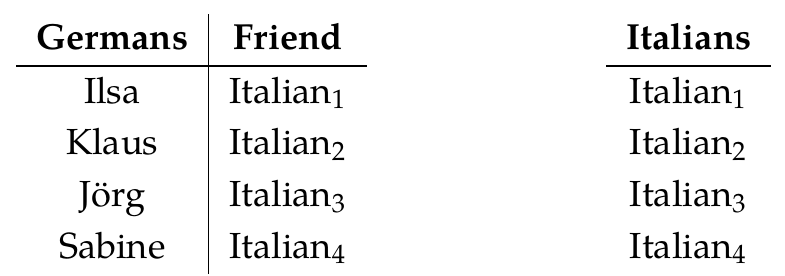In short, it makes up placeholders with arbitrary names to fill in the missing data. And it does this in a very systematic way. It doesn't add unnecessary equations, like giving Jörg and Sabine the same friend. And it doesn't add unnecessary elements, like some guy $$\mathrm{Italian}_7$$ who is not anybody's friend!

This is useful not because it gives us the 'right answer' to the impossible problem of guessing information about Italians when all we know about is the Germans. It's useful because it gives us an acceptable, systematically constructed database that we can then update as we learn more information.

The cool part is that all this follows from the definition of 'left adjoint'.

In other words, there's a natural one-to-one correspondence between

$$\textrm{ morphisms from } \textrm{Lan}_G(H) \textrm{ to } F$$ in the category $$\mathbf{Set}^\mathcal{C}$$ and

$$\textrm{morphisms from } H \textrm{ to } F \circ G$$ in the category $$\mathbf{Set}^\mathcal{D}$$.

Remember what these morphisms are: they are morphisms between functors, so they are natural transformations. Databases are functors, and we saw what natural transformations between them look like in Puzzles 127-128 and Puzzle 148.

So I'm saying that there's a natural one-to-one correspondence between

$$\textrm{ natural transformations } \alpha: \textrm{Lan}_G(H) \Rightarrow F$$ and

$$\textrm{natural transformations } \beta: H \Rightarrow F \circ G$$ This is true, not just for my particular example of $$F$$ and $$H$$, but for any example. But let's think about it in my example.

Puzzle 155. Describe a natural transformation $$\alpha : \textrm{Lan}_G(H) \Rightarrow F$$ where $$\textrm{Lan}_G(H)$$ is this database:and $$F$$ is this database:Puzzle 156. Describe a natural transformation $$\beta: H \Rightarrow F \circ G$$ where $$H$$ is this database:and $$F \circ G$$ is this database:Puzzle 157. Which natural transformation $$\beta: H \Rightarrow F \circ G$$ corresponds to the natural transformation $$\alpha :\textrm{Lan}_G(H) \Rightarrow F$$ you gave in Puzzle 155?

Puzzle 158. How many natural transformations from $$\textrm{Lan}_G(H)$$ to $$F$$ are there? How many natural transformations from $$H$$ to $$F \circ G$$ are there?

You'd better get the same answer to both parts of Puzzle 158, since there's supposed to be a one-to-one correspondence between these natural transformations! However, it's best to think about both questions separately and see why you're getting the same answer!

Finally:

Puzzles 159. The categories I've been calling $$\mathcal{C}$$ and $$\mathcal{D}$$ have other, more purely mathematical names. More precisely, they are isomorphic to two other categories we've already seen in this course, which have more mathematical names. What are those other categories?

To read other lectures go here.

• Options
1.
edited June 2018

Puzzle 155 Well let's be a bit silly and take all the germans to Heinrich, then naturality forces us to take all the italians to Martina.

Puzzle 156 Because there's no multicolumn table, aka function, aka morphism in Set, any function at all from {Ilsa, Klaus, Jorg, Sabine} to {Ilsa, Klaus, Jorg, Sabine, Heinrich} defines a natural transformation.

And now it all clicks for me. Huh.

Comment Source:**Puzzle 155** Well let's be a bit silly and take all the germans to Heinrich, then naturality forces us to take all the italians to Martina. **Puzzle 156** Because there's no multicolumn table, aka function, aka morphism in Set, any function at all from {Ilsa, Klaus, Jorg, Sabine} to {Ilsa, Klaus, Jorg, Sabine, Heinrich} defines a natural transformation. And now it all clicks for me. Huh. 
• Options
2.
edited June 2018

Great, Christopher!

Now someone please tackle Puzzle 157, because this is where we start understanding left Kan extensions!

Comment Source:Great, Christopher! Now someone please tackle Puzzle 157, because this is where we start understanding left Kan extensions!
• Options
3.

I think I won't give a lecture today, because I feel people need to catch up with what I've already done. If you can't do the puzzles in the above lecture, please ask me questions!

Comment Source:I think I won't give a lecture today, because I feel people need to catch up with what I've already done. If you can't do the puzzles in the above lecture, please ask me questions!
• Options
4.
edited June 2018

Puzzle 157: Using Christopher's answer to 156 we should get the natural transformation which sends every German to Heinrich. The idea is that a natural transformation $$\alpha : \mathrm{Lan}_G (H) \rightarrow F$$ is uniquely determined by where it sends the Germans because naturality will always give a unique map for the Italians. This map which $$\alpha$$ is uniquely determined by should be the map $$\beta$$ because there is a bijection between such maps from the adjunction equations.

Puzzle 158: I'll start by answering the second part because it is easier. Because there are no naturality conditions to check, this number should be the number of functions between the Germans in the image of $$F$$ to the Germans in the image of $$H$$. That number should be $$|H(\mathrm{Germans})|^{|F(\mathrm{Germans})|} = 5^4$$. To find the number of natural transformations $$\alpha : \mathrm{Lan}_G (H) \rightarrow F$$ we note that by naturality of $$\alpha$$, $$\alpha$$ is uniquely determined by its function from the germans in $$\mathrm{Lan}_G (H)$$ to the Germans in $$F$$. So this number should also be $$5^4$$.

Puzzle 159: Is it the arrow category and the terminal category respectively?

Comment Source:Puzzle 157: Using Christopher's answer to 156 we should get the natural transformation which sends every German to Heinrich. The idea is that a natural transformation \$$\alpha : \mathrm{Lan}_G (H) \rightarrow F \$$ is uniquely determined by where it sends the Germans because naturality will always give a unique map for the Italians. This map which \$$\alpha\$$ is uniquely determined by should be the map \$$\beta\$$ because there is a bijection between such maps from the adjunction equations. Puzzle 158: I'll start by answering the second part because it is easier. Because there are no naturality conditions to check, this number should be the number of functions between the Germans in the image of \$$F\$$ to the Germans in the image of \$$H\$$. That number should be \$$|H(\mathrm{Germans})|^{|F(\mathrm{Germans})|} = 5^4\$$. To find the number of natural transformations \$$\alpha : \mathrm{Lan}_G (H) \rightarrow F\$$ we note that by naturality of \$$\alpha\$$, \$$\alpha\$$ is uniquely determined by its function from the germans in \$$\mathrm{Lan}_G (H)\$$ to the Germans in \$$F\$$. So this number should also be \$$5^4\$$. Puzzle 159: Is it the arrow category and the terminal category respectively?
• Options
5.

I'll take a stab at puzzle 157.

Puzzle 157. Which natural transformation $$\beta: H \Rightarrow F \circ G$$ corresponds to the natural transformation $$\alpha :\textrm{Lan}_G(H) \Rightarrow F$$ you gave in Puzzle 155?

I think that given a natural transformation $$\alpha$$, we can obtain a natural transformation $$\beta$$ by inheriting the "Germans" component, that is $$\beta_{\mathrm{Germans}} = \alpha_{\mathrm{Germans}}$$. For Christopher's example, from the first comment, the natural transformation $$\beta$$ would be:

$\beta_{\mathrm{Germans}} = \left\{ \mathrm{Ilsa} \mapsto \mathrm{Heinrich}, \mathrm{Klaus} \mapsto \mathrm{Heinrich}, \mathrm{Jorg} \mapsto \mathrm{Heinrich}, \mathrm{Sabine} \mapsto \mathrm{Heinrich} \right\}$

However, I'm not sure how I can obtain this sort of correspondence for the general case: given a pair of adjoint functors $$F \dashv G$$, I understand that there exists a one-to-one correspondence between $$\mathcal{D}(F(A), B)$$ and $$\mathcal{C}(A, G(B))$$, but I don't know how to get a hold on the bijection $$\phi : \mathcal{D}(F(A), B) \to \mathcal{C}(A, G(B))$$.

Comment Source:I'll take a stab at puzzle 157. > **Puzzle 157.** Which natural transformation \$$\beta: H \Rightarrow F \circ G\$$ corresponds to the natural transformation \$$\alpha :\textrm{Lan}\_G(H) \Rightarrow F\$$ you gave in Puzzle 155? I think that given a natural transformation \$$\alpha\$$, we can obtain a natural transformation \$$\beta\$$ by inheriting the "Germans" component, that is \$$\beta_{\mathrm{Germans}} = \alpha_{\mathrm{Germans}}\$$. For Christopher's example, from [the first comment](https://forum.azimuthproject.org/discussion/comment/19649/#Comment_19649), the natural transformation \$$\beta\$$ would be: \$\beta_{\mathrm{Germans}} = \left\\{ \mathrm{Ilsa} \mapsto \mathrm{Heinrich}, \mathrm{Klaus} \mapsto \mathrm{Heinrich}, \mathrm{Jorg} \mapsto \mathrm{Heinrich}, \mathrm{Sabine} \mapsto \mathrm{Heinrich} \right\\} \$ However, I'm not sure how I can obtain this sort of correspondence for the general case: given a pair of adjoint functors \$$F \dashv G\$$, I understand that there exists a one-to-one correspondence between \$$\mathcal{D}(F(A), B)\$$ and \$$\mathcal{C}(A, G(B))\$$, but I don't know how to get a hold on the bijection \$$\phi : \mathcal{D}(F(A), B) \to \mathcal{C}(A, G(B))\$$.
• Options
6.
edited June 2018

Puzzles 159. The categories I've been calling $$\mathcal{C}$$ and $$\mathcal{D}$$ have other, more purely mathematical names. More precisely, they are isomorphic to two other categories we've already seen in this course, which have more mathematical names. What are those other categories?

I think $$\mathcal{C}$$ has been called "$$\mathbf{2}$$" in other discussions and $$\mathcal{D}$$ has been called "$$\mathbf{1}$$".

Comment Source:> **Puzzles 159.** The categories I've been calling \$$\mathcal{C}\$$ and \$$\mathcal{D}\$$ have other, more purely mathematical names. More precisely, they are _isomorphic_ to two other categories we've already seen in this course, which have more mathematical names. What are those other categories? I think \$$\mathcal{C}\$$ has been called "\$$\mathbf{2}\$$" in other discussions and \$$\mathcal{D}\$$ has been called "\$$\mathbf{1}\$$".
• Options
7.
edited June 2018

re this:

As usual, the left adjoint is 'liberal' or 'generous'. It says "We have to give each German an Italian friend? Okay, let's give them each their own friend!"

Would it be right to say $$\textrm{Lan}_G(H)$$ is the free $$\mathcal{C}$$-database extending the $$\mathcal{D}$$-database $$H$$?

And that $$F\circ G$$ is the underlying $$\mathcal{D}$$-database of the $$\mathcal{C}$$-database $$F$$?

Comment Source:re this: > As usual, the left adjoint is 'liberal' or 'generous'. It says "We have to give each German an Italian friend? Okay, let's give them each their own friend!" Would it be right to say \$$\textrm{Lan}_G(H)\$$ is the **free** \$$\mathcal{C}\$$-database extending the \$$\mathcal{D}\$$-database \$$H\$$? And that \$$F\circ G\$$ is the **underlying** \$$\mathcal{D}\$$-database of the \$$\mathcal{C}\$$-database \$$F\$$?
• Options
8.

Anindya - yes, exactly! For those used to the terms 'free' and 'underlying', this way of talking sheds a lot of light on what's going on here.

Comment Source:Anindya - yes, exactly! For those used to the terms 'free' and 'underlying', this way of talking sheds a lot of light on what's going on here.
• Options
9.
edited June 2018

Dan wrote:

However, I'm not sure how I can obtain this sort of correspondence for the general case: given a pair of adjoint functors $$F \dashv G$$, I understand that there exists a one-to-one correspondence between $$\mathcal{D}(F(A), B)$$ and $$\mathcal{C}(A, G(B))$$, but I don't know how to get a hold on the bijection $$\phi : \mathcal{D}(F(A), B) \to \mathcal{C}(A, G(B))$$.

A pair of adjoint functors is, by definition, a pair of functors equipped with a bijection of this sort, which is also required to be natural:

Definition. Given categories $$\mathcal{A}$$ and $$\mathcal{B}$$, an adjunction is pair of functors $$F: \mathcal{A} \to \mathcal{B}$$ and $$G: \mathcal{B} \to \mathcal{A}$$ together with a natural isomorphism

$\phi : \mathcal{B}(F(-),-) \to \mathcal{A}(-,G(-)).$ We call $$F$$ the left adjoint of $$G$$, and $$G$$ the right adjoint of $$F$$.

So, it's not just that there exists a one-to-one correspondence between $$\mathcal{D}(F(A), B)$$ and $$\mathcal{C}(A, G(B))$$: rather, we only call $$F$$ and $$G$$ adjoint functors only if they are equipped with a one-to-one correspondence which is natural. So, nobody can justly claim to have a pair of adjoint functors until they can hand you this bijection. It is not up to you to find it; it is up to them to provide it!

I could be missing the point of your concern - but if not you, surely some others need to think hard about the difference between structure (like being equipped with) and property (like there exists).

One way I could be missing your point is this. Maybe you're more concerned about how you find adjunctions in the first place.

If we are trying to find a pair of adjoint functors between two categories, it won't just fall from the sky: we have to work for it. Usually we start by guessing two functors $$F: \mathcal{A} \to \mathcal{B}$$ and $$G: \mathcal{B} \to \mathcal{A}$$ that 'feel like adjoints' - for example, $$F$$ feels like it's freely building objects of $$\mathcal{B}$$ from objects of $$\mathcal{A}$$, while $$G$$ feels it's forgetting structure on objects of $$\mathcal{B}$$ to get objects of $$\mathcal{A}$$. That's true in this lecture's puzzles.

But then we have to dream up a bijection

$$\phi_{A,B} : \mathcal{D}(F(A), B) \to \mathcal{C}(A, G(B))$$ for every object $$A$$ of $$\mathcal{A}$$ and every object $$B$$ of $$\mathcal{B}$$. This is the key step. Usually we do this by studying $$\mathcal{D}(F(A), B)$$ and $$\mathcal{C}(A, G(B))$$ and realizing that they are 'two ways of thinking about the same thing'.

And then - the most technical part, which we haven't dug into yet - we have to prove that $$\phi$$ is natural. Luckily, this last part is just a calculation, which almost always works if our construction of it is 'systematic', not ad-hoc or requiring case-by-case choices.

Comment Source:Dan wrote: > However, I'm not sure how I can obtain this sort of correspondence for the general case: given a pair of adjoint functors \$$F \dashv G\$$, I understand that there exists a one-to-one correspondence between \$$\mathcal{D}(F(A), B)\$$ and \$$\mathcal{C}(A, G(B))\$$, but I don't know how to get a hold on the bijection \$$\phi : \mathcal{D}(F(A), B) \to \mathcal{C}(A, G(B))\$$. A pair of adjoint functors is, by definition, a pair of functors _equipped with_ a bijection of this sort, which is also required to be natural: > **Definition.** Given categories \$$\mathcal{A}\$$ and \$$\mathcal{B}\$$, an **adjunction** is pair of functors \$$F: \mathcal{A} \to \mathcal{B}\$$ and \$$G: \mathcal{B} \to \mathcal{A}\$$ together with a natural isomorphism > \$\phi : \mathcal{B}(F(-),-) \to \mathcal{A}(-,G(-)). \$ We call \$$F\$$ the **left adjoint** of \$$G\$$, and \$$G\$$ the **right adjoint** of \$$F\$$. So, it's not just that _there exists_ a one-to-one correspondence between \$$\mathcal{D}(F(A), B)\$$ and \$$\mathcal{C}(A, G(B))\$$: rather, we only call \$$F\$$ and \$$G\$$ adjoint functors only if they are _equipped with_ a one-to-one correspondence which is natural. So, nobody can justly claim to have a pair of adjoint functors until they can hand you this bijection. It is not up to you to find it; it is up to them to provide it! I could be missing the point of your concern - but if not you, surely some others need to think hard about the difference between structure (like _being equipped with_) and property (like _there exists_). One way I could be missing your point is this. Maybe you're more concerned about how you find adjunctions in the first place. If we are trying to _find_ a pair of adjoint functors between two categories, it won't just fall from the sky: we have to work for it. Usually we start by guessing two functors \$$F: \mathcal{A} \to \mathcal{B}\$$ and \$$G: \mathcal{B} \to \mathcal{A}\$$ that 'feel like adjoints' - for example, \$$F\$$ feels like it's freely building objects of \$$\mathcal{B}\$$ from objects of \$$\mathcal{A}\$$, while \$$G\$$ feels it's forgetting structure on objects of \$$\mathcal{B}\$$ to get objects of \$$\mathcal{A}\$$. That's true in this lecture's puzzles. But then we have to dream up a bijection $\phi_{A,B} : \mathcal{D}(F(A), B) \to \mathcal{C}(A, G(B))$ for every object \$$A\$$ of \$$\mathcal{A}\$$ and every object \$$B\$$ of \$$\mathcal{B}\$$. This is the key step. Usually we do this by studying \$$\mathcal{D}(F(A), B)\$$ and \$$\mathcal{C}(A, G(B)) \$$ and realizing that they are 'two ways of thinking about the same thing'. And then - the most technical part, which we haven't dug into yet - we have to prove that \$$\phi\$$ is natural. Luckily, this last part is just a calculation, which almost always works if our construction of it is 'systematic', not ad-hoc or requiring case-by-case choices. 
• Options
10.

If you formalize 'systemic construction' correctly then that almost always becomes always.

This is a very useful trick that gives a bit of extra strength to some type systems in programming. By constraining your self to the natural functions the correct implementation is often obvious, sometimes trivial.

Comment Source:If you formalize 'systemic construction' correctly then that almost always becomes always. This is a very useful trick that gives a bit of extra strength to some type systems in programming. By constraining your self to the natural functions the correct implementation is often obvious, sometimes trivial. 
• Options
11.
edited June 2018

Puzzle 159. The categories I've been calling $$\mathcal{C}$$ and $$\mathcal{D}$$ have other, more purely mathematical names. More precisely, they are isomorphic to two other categories we've already seen in this course, which have more mathematical names. What are those other categories?

Matthew wrote:

I think $$\mathcal{C}$$ has been called "$$\mathbf{2}$$" in other discussions and $$\mathcal{D}$$ has been called "$$\mathbf{1}$$".

That's right! They're part of a sequence of important categories, which are actually posets: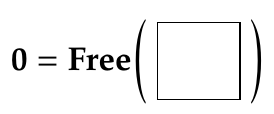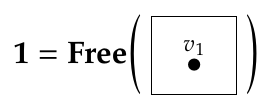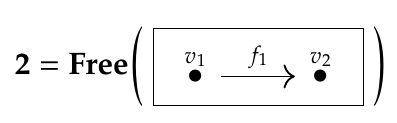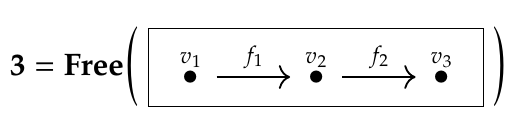and so on.

Puzzle 159: Is it the arrow category and the terminal category respectively?

That's also write! In previous puzzles we saw that:

• A functor $$F: \mathbf{1} \to \mathcal{A}$$ is the same as an object of the category $$\mathcal{A}$$.

• A functor $$F: \mathbf{2} \to \mathcal{A}$$ is the same as an arrow, or morphism, in the category $$\mathcal{A}$$,

• There is exactly one functor $$F: \mathcal{A} \to \mathbf{1}$$. Thus, we call $$\mathbf{1}$$ the terminal category.

• There is exactly one functor $$F :\mathbf{0} \to \mathcal{A}$$. Thus, we call $$\mathbf{0}$$ the initial category.

I didn't introduce the terms 'initial' and 'terminal', but we should!

Comment Source:> **Puzzle 159.** The categories I've been calling \$$\mathcal{C}\$$ and \$$\mathcal{D}\$$ have other, more purely mathematical names. More precisely, they are _isomorphic_ to two other categories we've already seen in this course, which have more mathematical names. What are those other categories? Matthew wrote: > I think \$$\mathcal{C}\$$ has been called "\$$\mathbf{2}\$$" in other discussions and \$$\mathcal{D}\$$ has been called "\$$\mathbf{1}\$$". That's right! They're part of a sequence of important categories, which are actually posets: <center><img src = "http://math.ucr.edu/home/baez/mathematical/7_sketches/category_0.png"></center> <center><img src = "http://math.ucr.edu/home/baez/mathematical/7_sketches/category_1.png"></center> <center><img src = "http://math.ucr.edu/home/baez/mathematical/7_sketches/category_2.png"></center> <center><img src = "http://math.ucr.edu/home/baez/mathematical/7_sketches/category_3.png"></center> and so on. Jade wrote: > Puzzle 159: Is it the arrow category and the terminal category respectively? That's also write! In previous puzzles we saw that: * A functor \$$F: \mathbf{1} \to \mathcal{A}\$$ is the same as an object of the category \$$\mathcal{A}\$$. * A functor \$$F: \mathbf{2} \to \mathcal{A}\$$ is the same as an arrow, or morphism, in the category \$$\mathcal{A}\$$, * There is exactly one functor \$$F: \mathcal{A} \to \mathbf{1} \$$. Thus, we call \$$\mathbf{1}\$$ the **terminal** category. * There is exactly one functor \$$F :\mathbf{0} \to \mathcal{A} \$$. Thus, we call \$$\mathbf{0}\$$ the **initial** category. I didn't introduce the terms 'initial' and 'terminal', but we should!
• Options
12.
edited June 2018

So, on to the main puzzles, Puzzles 155-158!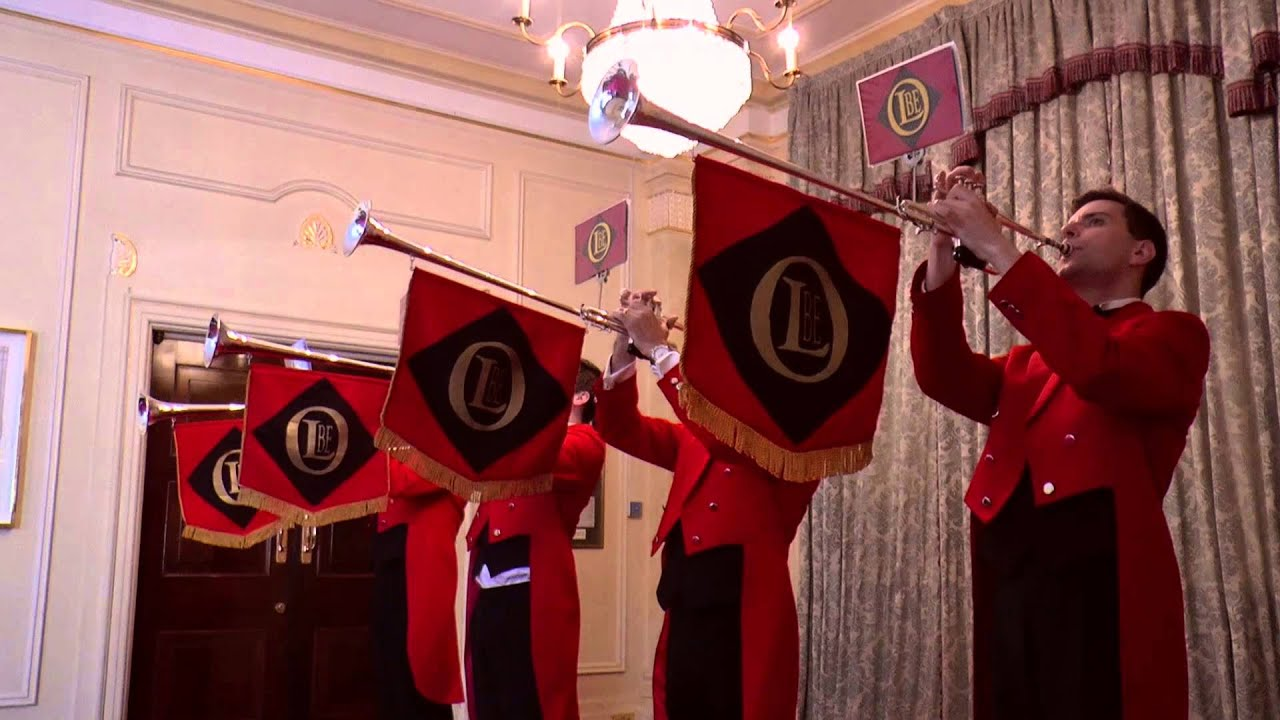I think you folks have mostly done them, but there's a bit left to do, and it's important for everyone to think about these, so let me start working through them very slowly and systematically - and everyone who has questions or comments jump in!

Puzzle 155. Describe a natural transformation $$\alpha : \textrm{Lan}_G(H) \Rightarrow F$$ where $$\textrm{Lan}_G(H)$$ is this database:and $$F$$ is this database:Both $$\textrm{Lan}_G(H)$$ and $$F$$ are functors from $$\mathcal{C}$$ to $$\mathbf{Set}$$, where $$\mathcal{C}$$ is the free category on this graph:So, a natural transformation $$\alpha : \textrm{Lan}_G(H) \Rightarrow F$$ consists of functions

$$\alpha_{\text{Germans}} : \textrm{Lan}_G(H)(\text{Germans}) \to F(\text{Germans})$$ and

$$\alpha_{\text{Italians}} : \textrm{Lan}_G(H)(\text{Italians}) \to F(\text{Italians}) .$$ Christopher proposed a choice for both of these:

Well let's be a bit silly and take all the Germans to Heinrich, then naturality forces us to take all the Italians to Martina.

By staring at the table above, note that

$$\textrm{Lan}_G(H)(\text{Germans}) = \{ \text{Ilsa}, \text{Klaus}, \mathrm{Jörg}, \text{Sabine} \}$$ and

$$F(\text{Germans}) = \{ \text{Ilsa}, \text{Klaus}, \text{Jörg}, \text{Sabine}, \text{Heinrich} \}.$$ So, we need to choose a function

$$\alpha_{\text{Germans}} : \{ \text{Ilsa}, \text{Klaus}, \text{Jörg}, \text{Sabine} \} \to \{ \text{Ilsa}, \text{Klaus}, \text{Jörg}, \text{Sabine} , \text{Heinrich} .\}$$ Christopher seems to be hinting that we can take this to be any function, and that naturality then forces a specific choice of the function

$$\alpha_{\text{Italians}} : \textrm{Lan}_G(H)(\text{Italians}) \to F(\text{Italians}) .$$ That's true. The key is the commutative square in the definition of natural transformation!

Could someone show, in a bit of detail, how this works? Some people instinctively 'get' the power of naturality. Others don't. My job is to make people get it, who don't yet. Maybe someone who is just starting to get it would enjoy filling in the details here!

Comment Source:So, on to the main puzzles, Puzzles 155-158! <center><img width = "300" src = "https://i.ytimg.com/vi/7Hujen-sFzQ/maxresdefault.jpg"></center> I think you folks have mostly done them, but there's a bit left to do, and it's important for everyone to think about these, so let me start working through them very slowly and systematically - and everyone who has questions or comments jump in! > **Puzzle 155.** Describe a natural transformation \$$\alpha : \textrm{Lan}_G(H) \Rightarrow F\$$ where \$$\textrm{Lan}_G(H) \$$ is this database: > <center><img width = "450" src = "http://math.ucr.edu/home/baez/mathematical/7_sketches/database_germans_friends_2.png"></center> > and \$$F\$$ is this database: > <center><img width = "480" src = "http://math.ucr.edu/home/baez/mathematical/7_sketches/database_germans_friends.png"></center> Both \$$\textrm{Lan}_G(H)\$$ and \$$F\$$ are functors from \$$\mathcal{C}\$$ to \$$\mathbf{Set}\$$, where \$$\mathcal{C}\$$ is the free category on this graph: <center><img width = "270" src = "http://math.ucr.edu/home/baez/mathematical/7_sketches/graph_database_5.png"></center> So, a natural transformation \$$\alpha : \textrm{Lan}_G(H) \Rightarrow F\$$ consists of functions $\alpha_{\text{Germans}} : \textrm{Lan}_G(H)(\text{Germans}) \to F(\text{Germans})$ and $\alpha_{\text{Italians}} : \textrm{Lan}_G(H)(\text{Italians}) \to F(\text{Italians}) .$ Christopher proposed a choice for both of these: > Well let's be a bit silly and take all the Germans to Heinrich, then naturality forces us to take all the Italians to Martina. By staring at the table above, note that $\textrm{Lan}_G(H)(\text{Germans}) = \\{ \text{Ilsa}, \text{Klaus}, \mathrm{J&ouml;rg}, \text{Sabine} \\}$ and $F(\text{Germans}) = \\{ \text{Ilsa}, \text{Klaus}, \text{J&ouml;rg}, \text{Sabine}, \text{Heinrich} \\}.$ So, we need to choose a function $\alpha_{\text{Germans}} : \\{ \text{Ilsa}, \text{Klaus}, \text{J&ouml;rg}, \text{Sabine} \\} \to \\{ \text{Ilsa}, \text{Klaus}, \text{J&ouml;rg}, \text{Sabine} , \text{Heinrich} .\\}$ Christopher seems to be hinting that we can take this to be _any_ function, and that naturality then _forces_ a specific choice of the function $\alpha_{\text{Italians}} : \textrm{Lan}_G(H)(\text{Italians}) \to F(\text{Italians}) .$ That's true. The key is the commutative square in the definition of [natural transformation](https://forum.azimuthproject.org/discussion/2244/lecture-43-chapter-3-natural-transformations/p1)! Could someone show, in a bit of detail, how this works? Some people instinctively 'get' the power of naturality. Others don't. My job is to make people get it, who don't yet. Maybe someone who is just starting to get it would enjoy filling in the details here!
• Options
13.

John – thank you for the clarifications from comment #9; they were all very valuable. My misunderstanding stemmed from the fact that I thought it's enough to show that there exists a bijection between the two hom-sets. This misunderstanding was reinforced by my solution to puzzle 150, which I now realize is incomplete since I didn't state explicitly what is the natural isomorphism between the two hom-sets. When I solved that puzzle I was thinking more in terms of cardinality of sets (if two sets have the same cardinality there is a bijection between them), but I was not concerned with choosing a particular bijection.

Comment Source:John – thank you for the clarifications from [comment #9](https://forum.azimuthproject.org/discussion/comment/19670/#Comment_19670); they were all very valuable. My misunderstanding stemmed from the fact that I thought it's enough to show that there exists a bijection between the two hom-sets. This misunderstanding was reinforced by [my solution](https://forum.azimuthproject.org/discussion/comment/19577/#Comment_19577) to puzzle 150, which I now realize is incomplete since I didn't state explicitly what is the natural isomorphism between the two hom-sets. When I solved that puzzle I was thinking more in terms of cardinality of sets (if two sets have the same cardinality there is a bijection between them), but I was not concerned with choosing a particular bijection.
• Options
14.
edited June 2018

Working on John's hint, puzzle 155 gives this commuting diagram.

$\begin{matrix} \text{Lan}_G(H)(\text{Germans}) & \overset{\text{Lan}_G(H)(\text{Friends})}\longrightarrow & \text{Lan}_G(H)(\text{Italians}) \\ \alpha_{\text{Germans}}\downarrow & & \downarrow\alpha_{\text{Italians}}\\ F(\text{Germans}) & \overset{F(\text{Friends})}\longrightarrow & F(\text{Italians}) \end{matrix}$

Comment Source:Working on John's hint, puzzle 155 gives this commuting diagram. \$\begin{matrix} \text{Lan}_G(H)(\text{Germans}) & \overset{\text{Lan}_G(H)(\text{Friends})}\longrightarrow & \text{Lan}_G(H)(\text{Italians}) \\\\ \alpha\_{\text{Germans}}\downarrow & & \downarrow\alpha\_{\text{Italians}}\\\\ F(\text{Germans}) & \overset{F(\text{Friends})}\longrightarrow & F(\text{Italians}) \end{matrix} \$
• Options
15.
edited June 2018

I want to make sure I get this...

So, given a functor $$G: \mathcal{D} \to \mathcal{C}$$, we can create a new functor from that acts by pre-composition, this functor acts backward to the original functor, like pullbacks did, so I'll call it $$G^*$$,

$G^* : [\mathcal{C},-] \to [\mathcal{D},-], \\ G^* = G\circ-$

then given another functor $$F : \mathcal{C} \to \mathbf{Set}$$, we can create a new functor,

$G^*F : [\mathcal{C},\mathbf{Set}] \to [\mathcal{D},\mathbf{Set}],$ so then $$H :\mathcal{D} \to \mathbf{Set}$$ is sort of like when there was a subset we wanted to look at, and $$\mathrm{Lan}_G(H)$$ is like the pullbacks left-adjoint (wasn't that an existential quantifier)?

I'm am getting this right, or am I torturing analogies here?

Comment Source:I want to make sure I get this... So, given a functor \$$G: \mathcal{D} \to \mathcal{C}\$$, we can create a new functor from that acts by pre-composition, this functor acts backward to the original functor, like pullbacks did, so I'll call it \$$G^*\$$, \$G^* : [\mathcal{C},-] \to [\mathcal{D},-], \\\\ G^* = G\circ- \$ then given another functor \$$F : \mathcal{C} \to \mathbf{Set}\$$, we can create a new functor, \$G^*F : [\mathcal{C},\mathbf{Set}] \to [\mathcal{D},\mathbf{Set}], \$ so then \$$H :\mathcal{D} \to \mathbf{Set}\$$ is sort of like when there was a subset we wanted to look at, and \$$\mathrm{Lan}_G(H)\$$ is like the pullbacks left-adjoint (wasn't that an existential quantifier)? I'm am getting this right, or am I torturing analogies here? 
• Options
16.
edited June 2018

Dan wrote:

When I solved that puzzle I was thinking more in terms of cardinality of sets (if two sets have the same cardinality there is a bijection between them), but I was not concerned with choosing a particular bijection.

Okay, it's good that we're clearing this up, then. This is one of many situations where having a specific bijection is much better than merely knowing one exists, which can be determined just by counting. For example, there's a big difference between knowing you have one wife and knowing who she is.

Comment Source:Dan wrote: > When I solved that puzzle I was thinking more in terms of cardinality of sets (if two sets have the same cardinality there is a bijection between them), but I was not concerned with choosing a particular bijection. Okay, it's good that we're clearing this up, then. This is one of many situations where having a specific bijection is much better than merely knowing one exists, which can be determined just by counting. For example, there's a big difference between knowing you have one wife and knowing who she is. 
• Options
17.
edited June 2018

Keith wrote:

So, given a functor $$G: \mathcal{D} \to \mathcal{C}$$, we can create a new functor from that acts by pre-composition, this functor acts backward to the original functor, like pullbacks did, so I'll call it $$G^*$$,

$G^* : [\mathcal{C},-] \to [\mathcal{D},-], \\ G^* = G\circ-$

You mean

$$G^* = - \circ G ,$$ because as you said, we are pre-composing with $$G$$, and we're using the usual notation where $$(F \circ G)(d) = F(G(d))$$ does $$G$$ first.

then given another functor $$F : \mathcal{C} \to \mathbf{Set}$$, we can create a new functor,

$G^*(F) : [\mathcal{C},\mathbf{Set}] \to [\mathcal{D},\mathbf{Set}],$ so then $$H :\mathcal{D} \to \mathbf{Set}$$ is sort of like when there was a subset we wanted to look at, and $$\mathrm{Lan}_G(H)$$ is like the pullback's left-adjoint (wasn't that an existential quantifier)?

Am I getting this right, or am I torturing analogies here?

No, everything you say here is right!

What I called $$F \circ G$$ is indeed often called the pullback of $$F$$ along $$G$$ and written $$F^\ast(G)$$. The left Kan extension functor $$\mathrm{Lan}_G$$ is the left adjoint of the pullback functor $$G^*$$.

And indeed, the left Kan extension is connected to existential quantifiers - though it's more closely connected to sums, which is why Fong and Spivak call it $$\sum$$. Back when we were studying preorders, also known as $$\textbf{Bool}$$-categories, we were often interested in just whether or not something is true. Now we're often interested in the set of ways in which something is true. For example, we don't just care about whether there is a German in our database: we want to know the actual set of Germans!

The only reason I've been writing stuff like

$$\textrm{composing with } G : \mathbf{Set}^{\mathcal{C}} \to \mathbf{Set}^{\mathcal{D}}$$

$$G^\ast : \mathbf{Set}^{\mathcal{C}} \to \mathbf{Set}^{\mathcal{D}}$$ is that I figure most people are already reeling under the onslaught of new notation and concepts. Congrats on seeing through this!

Comment Source:Keith wrote: > So, given a functor \$$G: \mathcal{D} \to \mathcal{C}\$$, we can create a new functor from that acts by pre-composition, this functor acts backward to the original functor, like pullbacks did, so I'll call it \$$G^*\$$, > \$G^* : [\mathcal{C},-] \to [\mathcal{D},-], \\\\ G^* = G\circ- \$ You mean $G^* = - \circ G ,$ because as you said, we are _pre_-composing with \$$G\$$, and we're using the usual notation where \$$(F \circ G)(d) = F(G(d))\$$ does \$$G\$$ _first_. > then given another functor \$$F : \mathcal{C} \to \mathbf{Set}\$$, we can create a new functor, > \$G^*(F) : [\mathcal{C},\mathbf{Set}] \to [\mathcal{D},\mathbf{Set}], \$ so then \$$H :\mathcal{D} \to \mathbf{Set}\$$ is sort of like when there was a subset we wanted to look at, and \$$\mathrm{Lan}_G(H)\$$ is like the pullback's left-adjoint (wasn't that an existential quantifier)? > Am I getting this right, or am I torturing analogies here? No, everything you say here is right! What I called \$$F \circ G\$$ is indeed often called the **pullback of \$$F\$$ along \$$G\$$** and written \$$F^\ast(G)\$$. The left Kan extension functor \$$\mathrm{Lan}_G\$$ is the left adjoint of the pullback functor \$$G^*\$$. And indeed, the left Kan extension is connected to existential quantifiers - though it's more closely connected to sums, which is why Fong and Spivak call it \$$\sum\$$. Back when we were studying preorders, also known as \$$\textbf{Bool}\$$-categories, we were often interested in just whether or not something is true. Now we're often interested in the _set of ways_ in which something is true. For example, we don't just care about whether there is a German in our database: we want to know the actual set of Germans! The only reason I've been writing stuff like > $\textrm{composing with } G : \mathbf{Set}^{\mathcal{C}} \to \mathbf{Set}^{\mathcal{D}}$ instead of $G^\ast : \mathbf{Set}^{\mathcal{C}} \to \mathbf{Set}^{\mathcal{D}}$ is that I figure most people are already reeling under the onslaught of new notation and concepts. Congrats on seeing through this!
• Options
18.
edited June 2018

Let's call this category $$\mathcal{D}$$. A functor $$F: \mathcal{C} \to \mathbf{Set}$$ is a database consisting of a set of Germans.

Is this correct? Shouldn't it be "A functor $$F: \mathcal{D} \to \mathbf{Set}$$ is a database consisting of a set of Germans"?

Comment Source:>Let's call this category \$$\mathcal{D}\$$. A functor \$$F: \mathcal{C} \to \mathbf{Set} \$$ is a database consisting of a set of Germans. Is this correct? Shouldn't it be "A functor \$$F: \mathcal{D} \to \mathbf{Set} \$$ is a database consisting of a set of Germans"?
• Options
19.
edited June 2018

Michael - thanks, I'll fix that typo! You're right. $$\mathcal{D}$$ is the free category on this graph:while $$\mathcal{C}$$ is the free category on this one:Comment Source:Michael - thanks, I'll fix that typo! You're right. \$$\mathcal{D}\$$ is the free category on this graph: <center><img width = "70" src = "http://math.ucr.edu/home/baez/mathematical/7_sketches/graph_database_6.png"></center> while \$$\mathcal{C}\$$ is the free category on this one: <center><img width = "200" src = "http://math.ucr.edu/home/baez/mathematical/7_sketches/graph_database_5.png"></center> I'm glad you're still here!
• Options
20.
edited June 2018

@John Lol I've been busy catching up. This stuff is great!

This first picture shows the relationship between all the functors and $$\mathrm{Lan}_{G}(H)$$ used in this Lecture.I can guess where to draw $$\mathrm{Ran}_{G}(H)$$ but don't know the details yet so I have left it out.

So now onto Puzzle 155-157. I used the most obvious example which is send each person to the same person and drew them out in full detail.The picture shows $$\beta:H \Rightarrow F \circ G$$ on the left and $$\alpha: \mathrm{Lan}_{G}(H) \Rightarrow F$$ on the right. The naturality square has become a naturality circle. You can follow using Keith's diagram in Comment 14. And as you can see $$\beta_{\mathrm{Germans}} = \alpha_{\mathrm{Germans}}$$.

Comment Source:@John Lol I've been busy catching up. This stuff is great! This first picture shows the relationship between all the functors and \$$\mathrm{Lan}_{G}(H)\$$ used in this Lecture. ![Left Kan Extension](http://aether.co.kr/images/left_kan_extension.svg) I can guess where to draw \$$\mathrm{Ran}_{G}(H)\$$ but don't know the details yet so I have left it out. So now onto **Puzzle 155-157**. I used the most obvious example which is send each person to the same person and drew them out in full detail. ![Puzzle 155-157](http://aether.co.kr/images/kan_extension_example.svg) The picture shows \$$\beta:H \Rightarrow F \circ G \$$ on the left and \$$\alpha: \mathrm{Lan}\_{G}(H) \Rightarrow F \$$ on the right. The naturality square has become a naturality circle. You can follow using Keith's diagram in Comment 14. And as you can see \$$\beta_{\mathrm{Germans}} = \alpha_{\mathrm{Germans}}\$$. 
• Options
21.
edited June 2018

Michael - very nice pictures! I especially like this one:At first I thought the blue arrow should point from $$\alpha$$ to $$\beta$$, because the definition of left Kan extension says there's a one-to-one and onto function from the set of

$$\text{ natural transformations } \alpha: \text{Lan}_G(H) \Rightarrow F$$ to the set of

$$\text{ natural transformation } \beta: H \Rightarrow F \circ G$$ Since it's one-to-one and onto, we could have a double-headed arrow pointing from $$\alpha$$ to $$\beta$$ and also back from $$\beta$$ to $$\alpha$$.

But then I realized your blue arrow pointing from $$H$$ to $$\text{Lan}_G (H)$$ is also a real thing, it's the process of left Kan extending along $$G$$, namely the functor

$$\text{Lan}_G : \mathbf{Set}^\mathcal{D} \to \mathbf{Set}^\mathcal{C} .$$ Whew, there are lots of arrows here!

Comment Source:Michael - very nice pictures! I especially like this one: <center><img src = "http://aether.co.kr/images/left_kan_extension.svg"></center> At first I thought the blue arrow should point from \$$\alpha\$$ to \$$\beta\$$, because the definition of left Kan extension says there's a one-to-one and onto function from the set of $\text{ natural transformations } \alpha: \text{Lan}_G(H) \Rightarrow F$ to the set of $\text{ natural transformation } \beta: H \Rightarrow F \circ G$ Since it's one-to-one and onto, we could have a double-headed arrow pointing from \$$\alpha\$$ to \$$\beta\$$ and also back from \$$\beta\$$ to \$$\alpha\$$. But then I realized your blue arrow pointing from \$$H\$$ to \$$\text{Lan}\_G (H) \$$ is also a real thing, it's the _process of left Kan extending along \$$G\$$_, namely the functor $\text{Lan}\_G : \mathbf{Set}^\mathcal{D} \to \mathbf{Set}^\mathcal{C} .$ Whew, there are lots of arrows here!
• Options
22.
edited June 2018

John

Cool! That's even nicer than I thought. I was having a hard time seeing how the Kan extension was working so I thats why drew blue arrow from $$H$$ to $$Lan_{G}(H)$$ to help me realize that its just a function from $$H$$ along $$G$$. The picture makes much more sense now with the one-to-one and onto function from $$\alpha$$ to $$\beta$$.Comment Source:John Cool! That's even nicer than I thought. I was having a hard time seeing how the Kan extension was working so I thats why drew blue arrow from \$$H\$$ to \$$Lan_{G}(H)\$$ to help me realize that its just a function from \$$H\$$ along \$$G\$$. The picture makes much more sense now with the one-to-one and onto function from \$$\alpha\$$ to \$$\beta\$$. ![Left Kan Extension with one-to-one function](http://aether.co.kr/images/left_kan_extension_2.svg)
• Options
23.
edited June 2018

Thinking about the example, and about what naturality means with the actual data, I started to think laterally and came to the suspicion that what was sought was Puzzle 151. I was thinking, the category of database $$\mathcal{C}$$-instances is $$\mathbf{Set}^\mathbf{2}$$, and the category of $$\mathcal{D}$$-instances is $$\mathbf{Set}^\mathbf{1}$$, i. e. (equivalent to), $$\mathbf{Set}$$. At the level of instances, forgetting italians would be the functor $$F: \mathbf{Set}^2 \to \mathbf{Set}$$ defined in the puzzle, and we seek a left adjoint. But I think there's a problem!

What is exactly "$$\mathbf{2}$$"? In puzzle 151 I think it is just two isolated identities. But here it is as in this comment. So the category of $$\mathcal{C}$$-instances is more exactly $$\mathbf{Set}^{\rightarrow}$$, the arrow category of Set, that is, the category of functions and commutative squares. Our functor of precomposing instances with $$G$$ is (in this example), I believe, the more sophisticated domain opfibration $$dom: \mathbf{Set}^{\rightarrow} \to \mathbf{Set}$$, so we are asking for a left adjoint to that. Looks hairy.

Comment Source:One concern also after a wiggly road ahead warning. Thinking about the example, and about what naturality means with the actual data, I started to think laterally and came to the suspicion that what was sought was [Puzzle 151](https://forum.azimuthproject.org/discussion/2264/lecture-48-chapter-3-adjoint-functors). I was thinking, the category of database \$$\mathcal{C}\$$-instances is \$$\mathbf{Set}^\mathbf{2}\$$, and the category of \$$\mathcal{D}\$$-instances is \$$\mathbf{Set}^\mathbf{1}\$$, i. e. (equivalent to), \$$\mathbf{Set}\$$. At the level of instances, forgetting italians would be the functor \$$F: \mathbf{Set}^2 \to \mathbf{Set}\$$ defined in the puzzle, and we seek a left adjoint. But I think there's a problem! What is exactly "\$$\mathbf{2}\$$"? In puzzle 151 I think it is just two isolated identities. But here it is as in [this comment](https://forum.azimuthproject.org/discussion/comment/19672/#Comment_19672). So the category of \$$\mathcal{C}\$$-instances is more exactly \$$\mathbf{Set}^{\rightarrow}\$$, the [arrow category](https://ncatlab.org/nlab/show/arrow+category) of **Set**, that is, the category of functions and commutative squares. Our functor of precomposing instances with \$$G\$$ is (in this example), I believe, the more sophisticated [domain opfibration](https://ncatlab.org/nlab/show/domain+opfibration) \$$dom: \\mathbf{Set}^{\rightarrow} \to \mathbf{Set}\$$, so we are asking for a left adjoint to **that**. Looks hairy.
• Options
24.
edited June 2018

Michael's diagram suggests that $$\textrm{Lan}_{G} (H) \circ G = H$$ which turns out to be the case. Is it true for left Kan extensions in general?

Comment Source:Michael's diagram suggests that \$$\textrm{Lan}_{G} (H) \circ G = H \$$ which turns out to be the case. Is it true for left Kan extensions in general?
• Options
25.
edited June 2018

I wouldn't say that $$\text{Lan}_{G} (H) \circ G = H$$ on the nose, but rather, $$\text{Lan}_{G} (H) \circ G \sim H$$.

In fact, $$\text{Lan}_{G} (H)$$ is a sort of pushforward operation, taking $$H : \mathcal{D} \to \mathbf{Set}$$ and $$\beta_\text{Germans} : H \to F$$, then pushing those forward along $$G : \mathcal{D} \to {C}$$.

$$H$$ here is acting like a subset of $$F\circ G$$ (in fact $$H$$ is a subfunctor of $$F \circ G$$) with $$\beta_\text{Germans}$$ a sort of inclusion natural transformation, and $$\textrm{Lan}_{G} (H)$$ is a pushforward of sorts along $$G$$.

Comment Source:I wouldn't say that \$$\text{Lan}\_{G} (H) \circ G = H\$$ on the nose, but rather, \$$\text{Lan}\_{G} (H) \circ G \sim H\$$. In fact, \$$\text{Lan}\_{G} (H) \$$ is a sort of pushforward operation, taking \$$H : \mathcal{D} \to \mathbf{Set}\$$ and \$$\beta\_\text{Germans} : H \to F\$$, then pushing those forward along \$$G : \mathcal{D} \to {C}\$$. \$$H\$$ here is acting like a subset of \$$F\circ G\$$ (in fact \$$H\$$ is a subfunctor of \$$F \circ G\$$) with \$$\beta_\text{Germans}\$$ a sort of inclusion natural transformation, and \$$\textrm{Lan}\_{G} (H)\$$ is a pushforward of sorts along \$$G\$$.
• Options
26.
edited June 2018

Michael's diagram suggests that $$\textrm{Lan}_{G} (H) \circ G = H$$ which turns out to be the case. Is it true for left Kan extensions in general?

I believe the answer, in general, is "No".

Let $$I_\mathcal{C}$$ be the identity functor. We have the following:

$$F \dashv \mathrm{Lan}_{F}\, I_\mathcal{C} \tag{A}$$ (see Bartosz Milewski's notes (2017) for more info on this)

In a Cartesian Closed Category, we have the following adjunction:

$$A \times (-) \dashv (-)^A \tag{B}$$ By (A) and (B), we have

$$\mathrm{Lan}_{A \times (-)}\, I_\mathcal{C} \cong (-)^A$$ This is because adjoints are unique up to isomorphism.

The composition $$(-)^A \circ A \times (-)$$ is a monad. In Haskell, it is commonly referred to as the State Monad - you can find details regarding this on nLab.

While the identity functor is a monad too, it is distinct from the state monad.

So we have:

$$(\mathrm{Lan}_{A \times (-)}\, I_\mathcal{C}) \circ (A \times (-)) \not\cong I_\mathcal{C}$$ This does lead me to another curiosity...

Puzzle MD1. If $$M$$ is a monad and $$\mathrm{Lan}_{M}\, I_\mathcal{C}$$ exists, then is it the case that $$(\mathrm{Lan}_{M}\, I_\mathcal{C}) \circ M \cong M$$?

Comment Source:@ [Anindya](https://forum.azimuthproject.org/discussion/comment/19702/#Comment_19702) > Michael's diagram suggests that \$$\textrm{Lan}_{G} (H) \circ G = H \$$ which turns out to be the case. Is it true for left Kan extensions in general? I believe the answer, in general, is "No". Let \$$I\_\mathcal{C}\$$ be the identity functor. We have the following: $F \dashv \mathrm{Lan}\_{F}\, I\_\mathcal{C} \tag{A}$ (see [Bartosz Milewski's notes (2017)](https://bartoszmilewski.com/2017/04/17/kan-extensions/) for more info on this) In a [Cartesian Closed Category](https://en.wikipedia.org/wiki/Cartesian_closed_category#Definition), we have the following adjunction: $A \times (-) \dashv (-)^A \tag{B}$ By (A) and (B), we have $\mathrm{Lan}\_{A \times (-)}\, I\_\mathcal{C} \cong (-)^A$ This is because adjoints are unique up to isomorphism. The composition \$$(-)^A \circ A \times (-) \$$ is a monad. In Haskell, it is commonly referred to as the [State Monad](https://wiki.haskell.org/State_Monad) - you can find details regarding this on [nLab](https://ncatlab.org/nlab/show/function+monad#relation_to_the_writer_comonad_and_state_monad). While the identity functor is a monad too, it is distinct from the state monad. So we have: $(\mathrm{Lan}\_{A \times (-)}\, I\_\mathcal{C}) \circ (A \times (-)) \not\cong I_\mathcal{C}$ This does lead me to another curiosity... **Puzzle MD1**. If \$$M\$$ is a monad and \$$\mathrm{Lan}\_{M}\, I\_\mathcal{C}\$$ exists, then is it the case that \$$(\mathrm{Lan}\_{M}\, I\_\mathcal{C}) \circ M \cong M\$$?
• Options
27.

@Matthew – cheers for that, I suspected the answer was no.

The adjunction tells us that there's a unit natural transformation $$\eta_H : H \Rightarrow \textrm{Lan}_G(H) \circ G$$ but there doesn't seem to be in general any reason to think this is a natural isomorphism, let alone an identity of functors.

@Keith – maybe I'm misunderstanding you but it seems to me that this particular example we do have $$\text{Lan}_{G} (H) \circ G = H$$ "on the nose" – the two functors agree on the single object of $$\mathcal{D}$$, do they not?

Comment Source:@Matthew – cheers for that, I suspected the answer was no. The adjunction tells us that there's a unit natural transformation \$$\eta_H : H \Rightarrow \textrm{Lan}_G(H) \circ G\$$ but there doesn't seem to be in general any reason to think this is a natural isomorphism, let alone an identity of functors. @Keith – maybe I'm misunderstanding you but it seems to me that this particular example we do have \$$\text{Lan}\_{G} (H) \circ G = H\$$ "on the nose" – the two functors agree on the single object of \$$\mathcal{D}\$$, do they not?
• Options
28.
edited June 2018

Puzzle MD1. If $$M$$ is a monad and $$\mathrm{Lan}_{M}\, I_\mathcal{C}$$ exists, then is it the case that $$(\mathrm{Lan}_{M}\, I_\mathcal{C}) \circ M \cong M$$?

Evidently if $$M$$ is a monad then $$\mathrm{Lan}_{M}\, I_\mathcal{C}$$, if it exists, is a comonad, according to nLab's entry on adjoint's monads.

Comment Source:> **Puzzle MD1**. If \$$M\$$ is a monad and \$$\mathrm{Lan}\_{M}\, I\_\mathcal{C}\$$ exists, then is it the case that \$$(\mathrm{Lan}\_{M}\, I\_\mathcal{C}) \circ M \cong M\$$? Okay, I am totally wrong about this. Evidently if \$$M\$$ is a monad then \$$\mathrm{Lan}\_{M}\, I\_\mathcal{C}\$$, if it exists, is a *comonad*, according to [nLab's entry on adjoint's monads](https://ncatlab.org/nlab/show/adjoint+monad).
• Options
29.
edited June 2018

Anindya wrote:

The adjunction tells us that there's a unit natural transformation $$\eta_H : H \Rightarrow \textrm{Lan}_G(H) \circ G$$ but there doesn't seem to be in general any reason to think this is a natural isomorphism...

Probably not in general, but sometimes! In the example of this lecture, it is. And I'm pretty sure we can generalize this fact to a bunch of similar situations. But to find these situations, we need to think about what goes wrong:

Puzzle. Find an functor $$G : \mathcal{D} \to \mathcal{C}$$ where $$\mathcal{C}$$ and $$\mathcal{D}$$ have just one object and very few morphisms, and it's not true that left Kan extending a functor $$H : \mathcal{D} \to \mathbf{Set}$$ along $$G$$ and then composing it with $$G$$ gets us back where started, up to natural isomorphism.

... let alone an identity of functors.

We should never care whether $$\textrm{Lan}_G(H) \circ G$$ is equal to $$H$$. The reason is that any functor naturally isomorphic to a left (or right) adjoint is again a left (or right) adjoint. So there's not really such a thing as 'the' left Kan extension functor $$\textrm{Lan}_G$$: any functor naturally isomorphic to this will also have all the properties in the definition of left Kan extension.

Comment Source:Anindya wrote: > The adjunction tells us that there's a unit natural transformation \$$\eta_H : H \Rightarrow \textrm{Lan}_G(H) \circ G\$$ but there doesn't seem to be in general any reason to think this is a natural isomorphism... Probably not in general, but sometimes! In the example of this lecture, it is. And I'm pretty sure we can generalize this fact to a bunch of similar situations. But to find these situations, we need to think about what goes wrong: **Puzzle.** Find an functor \$$G : \mathcal{D} \to \mathcal{C}\$$ where \$$\mathcal{C}\$$ and \$$\mathcal{D}\$$ have just one object and very few morphisms, and it's _not_ true that left Kan extending a functor \$$H : \mathcal{D} \to \mathbf{Set} \$$ along \$$G\$$ and then composing it with \$$G\$$ gets us back where started, up to natural isomorphism. > ... let alone an identity of functors. We should never care whether \$$\textrm{Lan}\_G(H) \circ G\$$ is _equal_ to \$$H\$$. The reason is that any functor naturally isomorphic to a left (or right) adjoint is again a left (or right) adjoint. So there's not really such a thing as 'the' left Kan extension functor \$$\textrm{Lan}_G\$$: any functor naturally isomorphic to this will also have all the properties in the definition of left Kan extension.
• Options
30.
edited June 2018

OK, let's take $$\mathcal{C}$$ to be the people/friend-of schema from Lecture 42.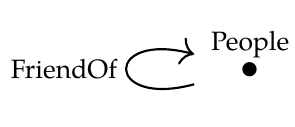And $$\mathcal{D}$$ is the same category but without the friend-of arrow. There's only one choice for $$G : \mathcal{D} \rightarrow \mathcal{C}$$, which is the obvious inclusion functor.

Now consider a very simple database $$H : \mathcal{D} \rightarrow \textbf{Set}$$ that sends the object of $$\mathcal{D}$$ to the set $$\{\textrm{Alice}\}$$. What is its left Kan extension $$\textrm{Lan}_G (H) : \mathcal{D} \rightarrow \textbf{Set}$$?

It has to send the object of $$\mathcal{C}$$ to a set $$P$$ of people, but also send the friend-of arrow in $$\mathcal{C}$$ to an endomap on $$P$$ – and do this in a "free" way.

That means we need $$\textrm{Alice} \in P$$, but then we need to invent a friend for her, say $$\textrm{Friend}_0 \in P$$, and a friend for him $$\textrm{Friend}_1 \in P$$, and a friend for her $$\textrm{Friend}_2 \in P$$...

So $$P$$ is a countably infinite set (isomorphic to the natural numbers). There's a natural inclusion map $$\{ \textrm{Alice} \} \rightarrow P$$ but these sets certainly aren't iso.

Hence $$\textrm{Lan}_G (H) \circ G$$ is *not* iso to $$H$$.

I'm guessing that the issue here is that the inclusion functor $$G$$ is not full.

Comment Source:OK, let's take \$$\mathcal{C}\$$ to be the people/friend-of schema from [Lecture 42](https://forum.azimuthproject.org/discussion/2236/lecture-42-chapter-3-transforming-databases). <center> <img width = "200" src = "http://math.ucr.edu/home/baez/mathematical/7_sketches/graph_database_4.png"></center> And \$$\mathcal{D}\$$ is the same category but without the friend-of arrow. There's only one choice for \$$G : \mathcal{D} \rightarrow \mathcal{C}\$$, which is the obvious inclusion functor. Now consider a very simple database \$$H : \mathcal{D} \rightarrow \textbf{Set}\$$ that sends the object of \$$\mathcal{D}\$$ to the set \$$\\{\textrm{Alice}\\}\$$. What is its left Kan extension \$$\textrm{Lan}_G (H) : \mathcal{D} \rightarrow \textbf{Set}\$$? It has to send the object of \$$\mathcal{C}\$$ to a set \$$P\$$ of people, but also send the friend-of arrow in \$$\mathcal{C}\$$ to an endomap on \$$P\$$ – and do this in a "free" way. That means we need \$$\textrm{Alice} \in P\$$, but then we need to invent a friend for her, say \$$\textrm{Friend}_0 \in P\$$, and a friend for him \$$\textrm{Friend}_1 \in P\$$, and a friend for her \$$\textrm{Friend}_2 \in P\$$... So \$$P\$$ is a countably infinite set (isomorphic to the natural numbers). There's a natural inclusion map \$$\\{ \textrm{Alice} \\} \rightarrow P\$$ but these sets certainly aren't iso. Hence \$$\textrm{Lan}_G (H) \circ G\$$ is *not* iso to \$$H\$$. I'm guessing that the issue here is that the inclusion functor \$$G\$$ is not full.
• Options
31.

(thinking about this further, if we flip round the roles of $$\mathcal{C}$$ and $$\mathcal{D}$$ above, we can show there's also an issue with functors that aren't faithful...)

Comment Source:(thinking about this further, if we flip round the roles of \$$\mathcal{C}\$$ and \$$\mathcal{D}\$$ above, we can show there's also an issue with functors that aren't faithful...)
• Options
32.
edited June 2018

Jesus wrote:

What is exactly "$$\mathbf{2}$$"? In puzzle 151 I think it is just two isolated identities. But here it is as in this comment. So the category of $$\mathcal{C}$$-instances is more exactly $$\mathbf{Set}^{\rightarrow}$$, the arrow category of Set, that is, the category of functions and commutative squares.

I've been using $$\mathbf{2}$$ with a boldface to mean the category you're calling $$\rightarrow$$, the category with two objects and one non-identity morphism. So $$\mathbf{Set}^{\mathbf{2}}$$ is just what you say: the arrow category of $$\mathbf{Set}$$, whose objects are functions and whose morphisms are commutative squares.

On the other hand, in Puzzle 151 I wrote $$\mathbf{Set}^2$$ - no boldface on the $$2$$ - to stand for the category $$\mathbf{Set} \times \mathbf{Set}$$, whose objects are pairs of sets and whose morphisms are pairs of functions. Sorry! It's hard to read the difference in font. But yes, if we wanted, we could use $$2$$ without a boldface to mean the category with two objects and only identity morphisms. Then $$\mathbf{Set}^2$$ would be another example of a functor category.

But yeah, it's bad use of notation to have $$\mathbf{Set}^{\mathbf{2}} \ne \mathbf{Set}^2$$.Comment Source:Jesus wrote: > What is exactly "\$$\mathbf{2}\$$"? In puzzle 151 I think it is just two isolated identities. But here it is as in [this comment](https://forum.azimuthproject.org/discussion/comment/19672/#Comment_19672). So the category of \$$\mathcal{C}\$$-instances is more exactly \$$\mathbf{Set}^{\rightarrow}\$$, the [arrow category](https://ncatlab.org/nlab/show/arrow+category) of **Set**, that is, the category of functions and commutative squares. I've been using \$$\mathbf{2}\$$ with a boldface to mean the category you're calling \$$\rightarrow\$$, the category with two objects and one non-identity morphism. So \$$\mathbf{Set}^{\mathbf{2}}\$$ is just what you say: the arrow category of \$$\mathbf{Set}\$$, whose objects are functions and whose morphisms are commutative squares. On the other hand, in Puzzle 151 I wrote \$$\mathbf{Set}^2\$$ - no boldface on the \$$2\$$ - to stand for the category \$$\mathbf{Set} \times \mathbf{Set}\$$, whose objects are pairs of sets and whose morphisms are pairs of functions. Sorry! It's hard to read the difference in font. But yes, if we wanted, we could use \$$2\$$ without a boldface to mean the category with two objects and only identity morphisms. Then \$$\mathbf{Set}^2\$$ would be another example of a functor category. But yeah, it's bad use of notation to have \$$\mathbf{Set}^{\mathbf{2}} \ne \mathbf{Set}^2\$$. <img src = "http://math.ucr.edu/home/baez/emoticons/redface.gif">
• Options
33.
edited June 2018

Anindya wrote:

K, let's take $$\mathcal{C}$$ to be the people/friend-of schema from Lecture 42.And $$\mathcal{D}$$ is the same category but without the friend-of arrow. There's only one choice for $$G : \mathcal{D} \rightarrow \mathcal{C}$$, which is the obvious inclusion functor.

Yes, that's the example I had in mind! $$\mathrm{Lan}_G$$ generates an infinite list of friends for any person, so if start with a database $$H$$ with just one person, then form $$\mathrm{Lan}_G(H)$$, then form $$\mathrm{Lan}_G(H) \circ G$$, we've got an enormous database with that person and an infinite list of others.

I'm guessing that the issue here is that the inclusion functor $$G$$ is not full.

That sounds about right. It would be fun to work out necessary and sufficient conditions.

Comment Source:Anindya wrote: > K, let's take \$$\mathcal{C}\$$ to be the people/friend-of schema from [Lecture 42](https://forum.azimuthproject.org/discussion/2236/lecture-42-chapter-3-transforming-databases). > <center> <img width = "200" src = "http://math.ucr.edu/home/baez/mathematical/7_sketches/graph_database_4.png"></center> > And \$$\mathcal{D}\$$ is the same category but without the friend-of arrow. There's only one choice for \$$G : \mathcal{D} \rightarrow \mathcal{C}\$$, which is the obvious inclusion functor. Yes, that's the example I had in mind! \$$\mathrm{Lan}_G\$$ generates an infinite list of friends for any person, so if start with a database \$$H\$$ with just one person, then form \$$\mathrm{Lan}_G(H)\$$, then form \$$\mathrm{Lan}_G(H) \circ G\$$, we've got an enormous database with that person and an infinite list of others. > I'm guessing that the issue here is that the inclusion functor \$$G\$$ is not full. That sounds about right. It would be fun to work out necessary and sufficient conditions. 
• Options
34.
edited June 2018

What if $$H$$ was an inclusion of $$\text{Italians}$$ instead of $$\text{Germans}$$?

How would $$\mathrm{Lan}_G(H)$$ behave then?

Comment Source:What if \$$H\$$ was an inclusion of \$$\text{Italians}\$$ instead of \$$\text{Germans}\$$? How would \$$\mathrm{Lan}\_G(H)\$$ behave then?
• Options
35.
edited June 2018

Keith - great question! I was thinking of posing that as a puzzle. Can you see how dramatically different it is?

(You can always compute an adjoint or Kan extension straight from the definition; it takes practice, but the best approach is to just dive in and try it.)

Comment Source:Keith - great question! I was thinking of posing that as a puzzle. Can you see how dramatically different it is? (You can always compute an adjoint or Kan extension straight from the definition; it takes practice, but the best approach is to just dive in and try it.) 
• Options
36.

Another approach to computing left and right Kan extensions is to use AQL :-). Below is AQL code to for the German/Italians example.


schema S = literal : empty { entities Germans }

schema T = literal : empty {
entities
Germans Italians
foreign_keys
friend : Germans -> Italians     }

mapping F = literal : S -> T {
entity Germans -> Germans }

instance I = literal : S {
generators
Ilsa Klaus Jorg Sabine : Germans
}

instance LanFI = sigma F I //left kan extension of I along F
instance FoLanFI = delta F LanFI //compose F with the left kan extension to get a monad

instance RanFI = pi F I //right kan extension of I along F

Comment Source:Another approach to computing left and right Kan extensions is to use AQL :-). Below is AQL code to for the German/Italians example. <pre> schema S = literal : empty { entities Germans } schema T = literal : empty { entities Germans Italians foreign_keys friend : Germans -> Italians } mapping F = literal : S -> T { entity Germans -> Germans } instance I = literal : S { generators Ilsa Klaus Jorg Sabine : Germans } instance LanFI = sigma F I //left kan extension of I along F instance FoLanFI = delta F LanFI //compose F with the left kan extension to get a monad transform monadUnit = unit F I //the unit of the adjunction instance RanFI = pi F I //right kan extension of I along F </pre>
• Options
37.

@John wrote:

It would be fun to work out necessary and sufficient conditions.

I'm having trouble with this. I've come up with a "hand-waving argument" about sufficient conditions, but can't quite see how to prove things rigorously using just the adjunction definition of the left Kan extension. I thought I'd write out the hand-waving version anyway to check that I'm on the right track more-or-less.

• if $$G$$ is full then the $$G$$-image of $$\mathcal{D}$$ in $$\mathcal{C}$$ is "self-contained" in the sense that there are no new arrows going out of the image and coming back in again. That means the "free" construction of $$\textrm{Lan}_G (H)$$ is well-behaved in the following sense: we are never forced to add new rows to the tables comprising $$H$$. So $$\eta_H$$ is surjective.

• if $$G$$ is faithful then the $$G$$-image of $$\mathcal{D}$$ in $$\mathcal{C}$$ is "lossless" in the sense that distinct $$\mathcal{D}$$-arrows remain distinct in the image. That means the "free" construction of $$\textrm{Lan}_G (H)$$ is never forces us to identify any rows in the tables comprising $$H$$. So $$\eta_H$$ is injective.

Putting these together we get $$G$$ full and faithful $$\implies \eta_H$$ bijective $$\implies \eta$$ iso.

Comment Source:@John wrote: > It would be fun to work out necessary and sufficient conditions. I'm having trouble with this. I've come up with a "hand-waving argument" about sufficient conditions, but can't quite see how to prove things rigorously using just the adjunction definition of the left Kan extension. I thought I'd write out the hand-waving version anyway to check that I'm on the right track more-or-less. • if \$$G\$$ is full then the \$$G\$$-image of \$$\mathcal{D}\$$ in \$$\mathcal{C}\$$ is "self-contained" in the sense that there are no new arrows going out of the image and coming back in again. That means the "free" construction of \$$\textrm{Lan}_G (H)\$$ is well-behaved in the following sense: we are never forced to add new rows to the tables comprising \$$H\$$. So \$$\eta_H\$$ is surjective. • if \$$G\$$ is faithful then the \$$G\$$-image of \$$\mathcal{D}\$$ in \$$\mathcal{C}\$$ is "lossless" in the sense that distinct \$$\mathcal{D}\$$-arrows remain distinct in the image. That means the "free" construction of \$$\textrm{Lan}_G (H)\$$ is never forces us to identify any rows in the tables comprising \$$H\$$. So \$$\eta_H\$$ is injective. Putting these together we get \$$G\$$ full and faithful \$$\implies \eta_H\$$ bijective \$$\implies \eta\$$ iso.
• Options
38.

Anindya - I agree that "full and faithful" should be sufficient; we could try to prove that sometime, though I bet people have already studied this. I keep changing my mind about whether "full" is necessary. I keep thinking that the real condition should be "no new arrows going out of the image and coming back in again". However, I now think that might be exactly the same as "full"!

Comment Source:Anindya - I agree that "full and faithful" should be sufficient; we could try to prove that sometime, though I bet people have already studied this. I keep changing my mind about whether "full" is necessary. I keep thinking that the real condition should be "no new arrows going out of the image and coming back in again". However, I now think that might be exactly the same as "full"!
• Options
39.

Puzzle Keith 50.1: What if G was an projection of Italians instead of Germans? How would $$Lan_G(H)$$ behave then? That is let G be the unique functor such that G(Italians)=Italians.

By the definition of Lan, forall H, there's a natural one-to-one correspondence between

$$\textrm{ morphisms from } \textrm{Lan}_G(H) \textrm{ to } F$$ in the category $$\mathbf{Set}^\mathcal{C}$$ and

$$\textrm{morphisms from } H \textrm{ to } F \circ G$$ in the category $$\mathbf{Set}^\mathcal{D}$$.

So let's say H maps "Italians" to {Alessandro, Martina, Bianca}.

So then morphisms from $$H \textrm{ to } F \circ G$$ are functions from {Alessandro, Martina, Bianca} to {Giuseppe, Giulia, Gian-Carlo, Alessandro, Martina, Bianca}. Just looking at cardinalities there are 6^3 of those, so there must also be 6^3 in morphisms from $$\textrm{Lan}_G(H) \textrm{ to } F$$ also. So the mapping from the germans must be determanied..but I think that forces the set of Germans to be the empty set in $$\textrm{Lan}_G(H)$$

Comment Source:Puzzle Keith 50.1: What if G was an projection of Italians instead of Germans? How would \$$Lan_G(H)\$$ behave then? That is let G be the unique functor such that G(Italians)=Italians. By the definition of Lan, forall H, there's a natural one-to-one correspondence between $\textrm{ morphisms from } \textrm{Lan}_G(H) \textrm{ to } F$ in the category \$$\mathbf{Set}^\mathcal{C}\$$ and $\textrm{morphisms from } H \textrm{ to } F \circ G$ in the category \$$\mathbf{Set}^\mathcal{D}\$$. So let's say H maps "Italians" to {Alessandro, Martina, Bianca}. So then morphisms from \$$H \textrm{ to } F \circ G \$$ are functions from {Alessandro, Martina, Bianca} to {Giuseppe, Giulia, Gian-Carlo, Alessandro, Martina, Bianca}. Just looking at cardinalities there are 6^3 of those, so there must also be 6^3 in morphisms from \$$\textrm{Lan}_G(H) \textrm{ to } F \$$ also. So the mapping from the germans must be determanied..but I think that forces the set of Germans to be the empty set in \$$\textrm{Lan}_G(H) \$$ 
• Options
40.
edited July 2018

JS3 I found this puzzle in some old notes on a paper by Joseph Goguen.

There is a unique choice of C (any category) for which the Yoneda embedding:

$$\mathcal{C} \to \mathbf{Set}^{\mathcal{C}^{\text{op}}}$$ has a string of four left adjoints.

What are they?

Comment Source:**JS3** I found this puzzle in some old notes on a paper by Joseph Goguen. There is a unique choice of C (any category) for which the Yoneda embedding: $\mathcal{C} \to \mathbf{Set}^{\mathcal{C}^{\text{op}}}$ has a string of four left adjoints. What are they?
• Options
41.
edited July 2018

Jim: I should know what you're talking about, that but I don't. In fact I don't believe that functor has an adjoint unless $$\mathcal{C}$$ is "sufficiently nice", e.g. a topos or something.

You didn't say what functor

$$\mathcal{C} \to \mathbf{Set}^{\mathcal{C}^{\text{op}}}$$ he's talking about; you can imagine various ones, but the best one is the Yoneda embedding. Everyone who wants to understand that should read this:

Comment Source:Jim: I should know what you're talking about, that but I don't. In fact I don't believe that functor has an adjoint unless \$$\mathcal{C}\$$ is "sufficiently nice", e.g. a topos or something. You didn't say what functor $\mathcal{C} \to \mathbf{Set}^{\mathcal{C}^{\text{op}}}$ he's talking about; you can imagine various ones, but the best one is the Yoneda embedding. Everyone who wants to understand that should read this: * Tae Danae-Bradley, [The Yoneda embedding](http://www.math3ma.com/mathema/2017/9/6/the-yoneda-embedding). 
• Options
42.
edited July 2018

I'm lagging a bit, but a question: I'm working to see the general idea that the German-Italian business exemplify, and seeking for a more systematic grip.

Would it be correct to say that in our example $$Lan_G$$ sends sets to identities? (or better, sends the things that correspond to sets in $$\mathbf{Set^1}$$ to an identity on that set?). Or better, since left adjoint functors are unique only up to natural isomorphism, that a functor obeying what I say is a left adjoint to $$- \circ G$$?

In more detail, be $$Ob(\mathbf{1})=\{\star\}$$, and $$Ob(\mathbf{2})=\{0, 1\}$$, $$Mor(\mathbf{2})=\{Id_0, Id_1, i:0 \to 1\}$$ and $$G(\star)=0$$.

Let $$S_1,S_2:\mathbf{1} \to \mathbf{Set}$$

$$Lan_G:\mathbf{Set}^\mathbf{1} \to \mathbf{Set}^\mathbf{2}$$ acts on objects and morphisms via functions

$$Lan_G^{Ob}: Ob(\mathbf{Set}^{\mathbf{1}}) \to Ob(\mathbf{Set}^{\mathbf{2}})$$ $$Lan_G^{Mor}: Mor(\mathbf{Set}^{\mathbf{1}}) \to Mor(\mathbf{Set}^{\mathbf{2}})$$ So if I submit a functor $$S_1$$ to $$Lan_G$$, I obtain a functor of $$\mathbf{Set}^{\mathbf{2}}$$.

I'am asking if it's OK to say that $$Lan_G$$ sends $$S_1$$ (and hence in a way its associated set $$S_1(\star)$$) to the identity on it, $$Id_{S_1(\star)}$$.

Yet more detailedly, can I say that $$Lan_G$$ on objects does this to $$S_1$$:

$$Lan_G^{Ob}(S_1)(0) = S_1(\star)$$ $$Lan_G^{Ob}(S_1)(1) = S_1(\star)$$ $$Lan_G^{Ob}(S_1)(i) = Id_{S_1(\star)}$$ and similarly for $$S_2$$

$$Lan_G^{Ob}(S_2)(0) = S_2(\star)$$ $$Lan_G^{Ob}(S_2)(1) = S_2(\star)$$ $$Lan_G^{Ob}(S_2)(i) = Id_{S_2(\star)}$$ ...and on arrows, for $$\mu:S_1 \Rightarrow S_2$$, so $$\mu \in hom_{\mathbf{Set}^\mathbf{1}}(S_1, S_2$$) with $$\mu_\star:S_1(\star) \to S_2(\star)$$, it assigns

$$Lan_G^{Mor}(\mu):=\tau$$ with $$\tau = \{\tau_0 := \mu_\star, \tau_1 := \mu_\star\}$$ ("duplicates arrows") soconmutes?

Comment Source:I'm lagging a bit, but a question: I'm working to see the general idea that the German-Italian business exemplify, and seeking for a more systematic grip. Would it be correct to say that in our example \$$Lan_G\$$ sends sets to identities? (or better, sends the things that correspond to sets in \$$\mathbf{Set^1}\$$ to an identity on that set?). Or better, since left adjoint functors are unique only up to natural isomorphism, that a functor obeying what I say is *a* left adjoint to \$$- \circ G\$$? In more detail, be \$$Ob(\mathbf{1})=\\{\star\\}\$$, and \$$Ob(\mathbf{2})=\\{0, 1\\}\$$, \$$Mor(\mathbf{2})=\\{Id_0, Id_1, i:0 \to 1\\}\$$ and \$$G(\star)=0\$$. Let \$$S_1,S_2:\mathbf{1} \to \mathbf{Set}\$$ \$$Lan_G:\mathbf{Set}^\mathbf{1} \to \mathbf{Set}^\mathbf{2}\$$ acts on objects and morphisms via functions $Lan_G^{Ob}: Ob(\mathbf{Set}^{\mathbf{1}}) \to Ob(\mathbf{Set}^{\mathbf{2}})$ $Lan_G^{Mor}: Mor(\mathbf{Set}^{\mathbf{1}}) \to Mor(\mathbf{Set}^{\mathbf{2}})$ So if I submit a functor \$$S_1\$$ to \$$Lan_G\$$, I obtain a functor of \$$\mathbf{Set}^{\mathbf{2}}\$$. I'am asking if it's OK to say that \$$Lan_G\$$ sends \$$S_1\$$ (and hence in a way its associated set \$$S_1(\star)\$$) to the identity on it, \$$Id_{S_1(\star)}\$$. Yet more detailedly, can I say that \$$Lan_G\$$ on objects does this to \$$S_1\$$: $Lan_G^{Ob}(S_1)(0) = S_1(\star)$ $Lan_G^{Ob}(S_1)(1) = S_1(\star)$ $Lan_G^{Ob}(S_1)(i) = Id_{S_1(\star)}$ and similarly for \$$S_2\$$ $Lan_G^{Ob}(S_2)(0) = S_2(\star)$ $Lan_G^{Ob}(S_2)(1) = S_2(\star)$ $Lan_G^{Ob}(S_2)(i) = Id_{S_2(\star)}$ ...and on arrows, for \$$\mu:S_1 \Rightarrow S_2\$$, so \$$\mu \in hom_{\mathbf{Set}^\mathbf{1}}(S_1, S_2\$$) with \$$\mu_\star:S_1(\star) \to S_2(\star)\$$, it assigns $Lan_G^{Mor}(\mu):=\tau$ with \$$\tau = \\{\tau_0 := \mu_\star, \tau_1 := \mu_\star\\}\$$ ("duplicates arrows") so ![naturality](http://i64.tinypic.com/vglx7n.jpg) conmutes? 
• Options
43.
edited July 2018

@John Re #40 Sorry I didn't follow the "specify what the whatsit is" rule. I've edited the post to try to correct it . Despite the page numbers in my notes I didn't note any link to the source and can't find it. I thought it was an interesting thing about a Yoneda embedding and hope the formulation is now ok.

Comment Source:@John Re #40 Sorry I didn't follow the "specify what the whatsit is" rule. I've edited the post to try to correct it . Despite the page numbers in my notes I didn't note any link to the source and can't find it. I thought it was an interesting thing about a Yoneda embedding and hope the formulation is now ok.
• Options
44.
edited July 2018

Christopher Upshaw wrote:

Puzzle Keith 50.1: What if G was an projection of Italians instead of Germans? How would $$Lan_G(H)$$ behave then? That is let G be the unique functor such that G(Italians)=Italians.

By the definition of Lan, forall H, there's a natural one-to-one correspondence between

$$\textrm{ morphisms from } \textrm{Lan}_G(H) \textrm{ to } F$$ in the category $$\mathbf{Set}^\mathcal{C}$$ and $$\textrm{morphisms from } H \textrm{ to } F \circ G$$ in the category $$\mathbf{Set}^\mathcal{D}$$.

So let's say H maps "Italians" to {Alessandro, Martina, Bianca}.

So then morphisms from $$H \textrm{ to } F \circ G$$ are functions from {Alessandro, Martina, Bianca} to {Giuseppe, Giulia, Gian-Carlo, Alessandro, Martina, Bianca}. Just looking at cardinalities there are 6^3 of those, so there must also be 6^3 in morphisms from $$\textrm{Lan}_G(H) \textrm{ to } F$$ also. So the mapping from the germans must be determanied..but I think that forces the set of Germans to be the empty set in $$\textrm{Lan}_G(H)$$

The set of Germans being empty seems reasonable, but I also got that we could simply ignore the names of the Germans and produce a placeholder if our Italian in question is a friend of some German. This also seems like a reasonable thing to do.

Comment Source:Christopher Upshaw wrote: >Puzzle Keith 50.1: What if G was an projection of Italians instead of Germans? How would \$$Lan_G(H)\$$ behave then? That is let G be the unique functor such that G(Italians)=Italians. >By the definition of Lan, forall H, there's a natural one-to-one correspondence between >$\textrm{ morphisms from } \textrm{Lan}_G(H) \textrm{ to } F$ >in the category \$$\mathbf{Set}^\mathcal{C}\$$ and >$\textrm{morphisms from } H \textrm{ to } F \circ G$ >in the category \$$\mathbf{Set}^\mathcal{D}\$$. >So let's say H maps "Italians" to {Alessandro, Martina, Bianca}. >So then morphisms from \$$H \textrm{ to } F \circ G \$$ are functions from {Alessandro, Martina, Bianca} to {Giuseppe, Giulia, Gian-Carlo, Alessandro, Martina, Bianca}. Just looking at cardinalities there are 6^3 of those, so there must also be 6^3 in morphisms from \$$\textrm{Lan}_G(H) \textrm{ to } F \$$ also. So the mapping from the germans must be determanied..but I think that forces the set of Germans to be the empty set in \$$\textrm{Lan}_G(H) \$$ The set of Germans being empty seems reasonable, but I also got that we could simply ignore the names of the Germans and produce a placeholder *if* our Italian in question is a friend of *some* German. This also seems like a reasonable thing to do. 
• Options
45.

Hmm. Yeah I am unsure what happens exactly.

Comment Source:Hmm. Yeah I am unsure what happens exactly.
• Options
46.

I'll answer this soon, Christopher! I gotta write a lecture now.

Comment Source:I'll answer this soon, Christopher! I gotta write a lecture now.
• Options
47.

Ooo! Looking forward to when you do!

Comment Source:Ooo! Looking forward to when you do!